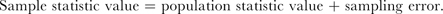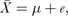This textbook offers an accessible and comprehensive introduction to statistics for all undergraduate psychology students, but particularly those in their second and third years who have already covered an initial introductory course. It covers all of the key areas in quantitative methods including sampling, significance tests, regression, and multivariate techniques and incorporates a range of exercises and problems at the end of each chapter for the student to follow.

The free CD-ROM with tutorial modules complements and enhances the exercises in the text, offers scope for distance learning, and makes both the traditional and non-traditional approaches much more accessible.

Key points of the book are: an emphasis on measurement, data summaries and graphs; a clear explanation of statistical inference using sampling distributions and confidence intervals, making significance tests much easier to understand; and help for students to understand and judge the use of particular tests in the research context beyond simple recipe following.

# Statistical Models and Significance Tests

### Statistical Models and Significance Tests

Statistical models and significance tests

### Statistical Models of a Population Statistic

In Chapter 5, we approached the concept of a confidence interval by starting with a statistical model of a sample statistic. This kind of model has the formA statistical model of a sample mean, for instance, when we know what the value of m is in advance, may be expressed bywhere e represents the sampling error in the model. In Chapter 5, we used the t-distribution as ...

•••